info@biomedres.us   +1 (720) 414-3554
One Westbrook Corporate Center, Suite 300, Westchester, IL 60154, USA

# Biomedical Journal of Scientific & Technical Research

September, 2019, Volume 22, 3, pp 16725-16729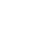Research Article

Research Article

# Discrete Models of Active Media in Attached to The Activities of Cardiac Arrhythmia

### Sergey Belyakin1* and Sergey Shuteev2

Author Affiliations

1Department of General Physics, Physics Faculty, Russia

2Laboratory of dynamic systems, Physics Faculty, Russia

Received: October 23, 2019 | Published: November 05, 2019

Corresponding author: Sergey Belyakin, Department of General Physics, Physics Faculty, Moscow, Russia

DOI: 10.26717/BJSTR.2019.22.003764

#### Abstract

This publication discusses models that describe the heart tissue as an active conductive system, considering its self-oscillating properties. In this type of models, cardiac rhythms can be described on the basis of the theory of dynamic systems, which justifies the need to build a universal model of oscillating medium. This type of model contributes to the understanding of these pathologies’ cardiac activity as parasecoli and AB-blockade.

#### Introduction

The first theoretical study of cardiac arrhythmias was conducted in 1920-ies of the van - der - Pol and van - der - Mark, who proposed an electrical model of the heart [1,2]. They suggested that the activity of the heart can be simulated by three nonlinear oscillators corresponding to ACS, Atria and ventricles. This possibility was confirmed by the fact that the graphical representation of the processes occurring in the system of nonlinear oscillators had a form similar to the action potentials of heart cells. In this system, there was a unidirectional connection between the sinus and atrial oscillators, and the same connection existed between the atrial and ventricular oscillators. Reducing the connection between the latter, they found that it is possible to obtain a number of different rhythms with phase captures, qualitatively corresponding to the ABblockades of the heart. After the publication of the work , many researchers tried to model the dynamics of heartbeats, believing it to be generated by several coupled oscillators. These developments can be divided into two large groups: analysis based on continuous - time representations (ODE systems) and studies based on discrete - time representations (finite-difference equations). In this section we will consider in detail the main stages of development of these concepts and the corresponding mathematical concepts.

#### Discrete Models & of A System of Interacting Pacemaker

Consider some physical quantity ξ, which reflects the internal state of the biological oscillator. Let own oscillator period is equal to T0. Let’s call a marker any event that can be clearly seen in the experiment, which is reached by the value ξ only once per period. Such a marker may be, for example, the beginning of the action potential in the cardiac preparation. Define the phase of the oscillator as follows. The phase of an arbitrarily selected marking event (for example, the maximum value of ξ) is assumed to be zero. At any next time, t, 0 < t < T0, the phase is defined as(mod1). Since the rhythm is restored after the perturbation of the system, the introduced phase completely determines the state of the system. Suppose that an external periodic perturbation acts on a nonlinear oscillator. Then each external influence shifts the state of the system to a new state: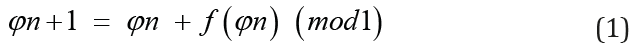The function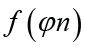is called the phase response curve (PRC) and determines the phase change after the stimulus. The points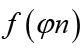of the system state are conveniently represented lying on the circle of the unit radius. Then, by iterating the mapping (1), one point of the circle is converted to another point of the same circle. If the circle map is continuous, then it can be characterized by a number called the topological degree and equal to the number of passes φn+1 on the unit circle for the time in which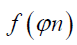passes it once.

In periodic perturbations of self-oscillations with a stable limit cycle, the dynamics is often described by maps of a circle with a topological degree 0 (when the over-threshold response gives rise to a new cycle) or 1 (which expresses a sub-threshold response to stimulation). The different types of circle maps are shown in Figure 1.

Figure 1: Different types of circle maps:
i. Reversible, topological degree 1
ii. Irreversible, topological degree 1
iii. Piecewise continuous
iv. Topological degree 0.

Along with the topological degree, an important characteristic of the circle display is the number of rotations. We define it as the time average ratio of the external perturbation period to the period of the perturbed oscillator. If the rotation number is rational, ρ = M/N (here M is the number of cycles of the stimulator, and N is the number of cycles of the nonlinear oscillator), then the dynamics of the system will be periodic with the capture of the phase of multiplicity N/M. If the rotation number is irrational, the system exhibits quasi-periodic or chaotic behavior. In many cases, the disturbance by a single pulse of a spontaneously oscillating system leads to a phase shift of the current rhythm (see, for example, [1,3] and references there). The magnitude of the shift depends on both the magnitude of the stimulus and its phase in the cycle. The graph of the dependence of the new phase on the previous phase (i.e., PRC) is either a continuous circle map with a topological degree of 1 or 0, or a discontinuous function. One of the simplest (and coarsest) approximations of a given PRC is the sinusoidal function, which ultimately results in a map of the form (2):Where a and b are constants. This approximation, for all its simplicity, correctly reflects the qualitative structure of the phase portrait of the system under study. The analysis of bifurcations of reversible circle maps was undertaken in the last century by A. Poincare and still attracts much -by V. Arnold  (see also  and the references given there). For pic.6 the bifurcation diagram of the circle diffeomorphism on the parameter plane (b, a) is shown. This diagram is divided into areas called language (or horns) of Arnold, which correspond to the sustainable capture phase ratio N/M (i.e., N cycles of the stimulator has M cycles of a nonlinear oscillator). Arnold languages exist for all rational relations N/M, where N and M are mutually Prime numbers. This means that there are an infinite number of Arnold languages that correspond to all possible ratios of frequencies of the stimulator and the perturbed oscillator. Between any two languages corresponding to N/M and N*/M* phase captures, there is another capture region corresponding to the capture of multiplicity phases (N+N*)/(M+M*). The structure shown in Figure 2, is the usual behavior for low stimulus amplitudes in simple theoretical models discussed below. However, as the amplitude of the periodic effect increases, this structure collapses.

Figure 2: Schematic diagram of Arnold tongues. In shaded areas there is a steady phase capture. There are always other zones between any two capture zones.

#### Model of Two Interacting Pacemakers Considering the Refractoriness Time

In this section, we consider two interacting leading centers (pulse oscillators) that can be pacemakers in cardiac tissue, construct a model of such interaction, and investigate its behavior.

### The principle of Constructing a Model

Consider a system of two interacting nonlinear pulse oscillators Figure 3. Let the momentum of the first oscillator with the period of undisturbed oscillations appear at the moment of time , and the momentum of the second oscillator with the period of undisturbed oscillations appear at the moment . Then the moments of time of occurrence of the following pulses are defined as:

Figure 3: The scheme of construction of the model describing the system of two interacting nonlinear oscillators.Now, assuming that under the influence of the second pulse, the period of the first oscillator will change by some value(where the expression in parentheses shows that this value depends only on the phase of the second pulse relative to the first), then the corresponding expression for tn+1 will look like:. When you consider that τn+1 > tn+1 , that for τn+1 get a similar expression: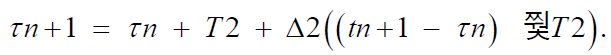. Dividing both of these expressions by T1, we find the corresponding expressions for the phases (3):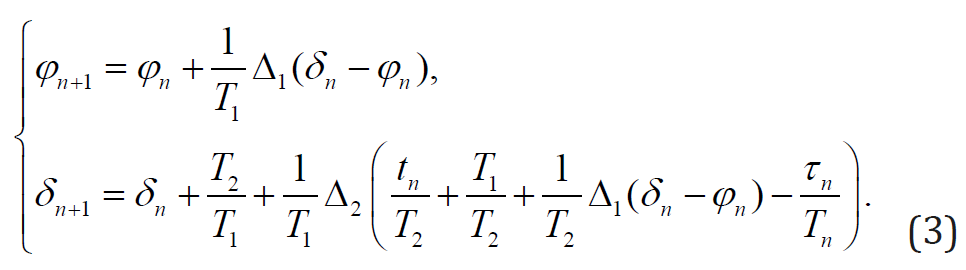Here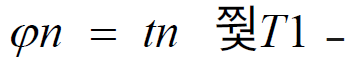phase of the first perturbed oscillator relative to the undisturbed (with the period T1),− the second phase of the disturbed oscillator with respect to the same first oscillation with a period of T1. Introducing the parameter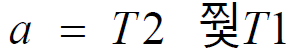(the ratio of the eigenfrequencies of both oscillators) and labeling f1 = Δ1 ∕ T1, f2 = Δ2 ∕ T1, after the transformations we obtain (4):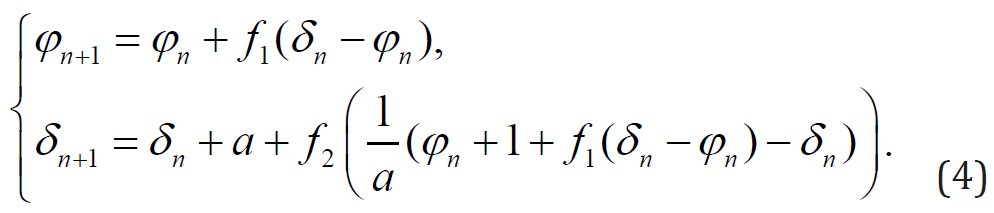Since we are interested in the phase difference of the described oscillators, the final expression, which will be used in the future, is as follows (5):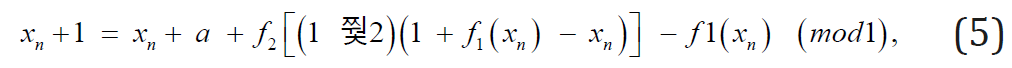where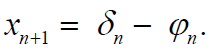Expressionincluded in the right side of the equation is a circle map describing the effect of the constant perturbation on the nonlinear oscillator. Considering the mutual influence of oscillators leads to the appearance of an additional nonlinear term. Thus:Function f1(x), f2(x) called phase response curves, which generally do not coincide with each other. Both oscillators are sources of action potentials in the same tissue, have a similar nature, and can be considered functions f1(x)and f2(x) approximately the same. It is known that the response of the oscillator to an external stimulus depends only on the stimulus phase of its amplitude and the PRC changes its shape when the amplitude of the external influence changes. This means that the functions that define the type of phase response curves must depend on one parameter that determines the magnitude of the amplitude. In the case of this dependence can be considered multiplicative. Then the phase response curves will be written as:Where h(x) − periodic function,Under this assumption, the formula (5) will take the form: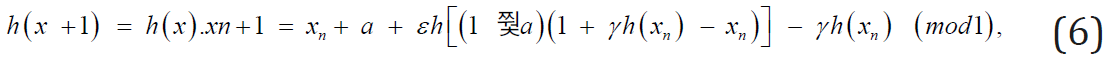Let’s focus on the study of the display (6) sinusoidal functions [6−8].

### Phase Diagrams for Systems with Mutual Influence

Let us now dwell on the case of two-way communication of two pulse systems. Suppose that the effect of the first oscillator on the second one is relatively small, ε = 0.1. As a PRC we will takeFor Figure 2 the corresponding phase diagram obtained as a result of numerical study is presented. It depicts for comparison the same steady grips phases ratio N:M. Here n cycles of the second oscillator account for M cycles of the first one. It is easy to see that considering the mutual influence of the oscillators leads to bending and splitting of the capture areas. Note also that even at small values of the amplitude of the second stimulus, the main captures are superimposed on each other. The dynamics of the system thus becomes multistable. This corresponds to the situation when the limiting behavior of the display (6) depends on the initial phase difference of the oscillators x0. For Figure 4 the circle map (6) constructed for some values of a and γ is given. It kind of confirms that with increasing γ the system dynamics becomes more complicated, and the display ceases to be monotonous, and it appears the intervals with the slope, a large 1. It can be shown  that for continuous circle maps this leads to multistability and chaotic dynamics.

Figure 4: Areas of stable phase captures for piecewise linear mapping of a circle (2) with a PRC of the form (6) considering the mutual influence of oscillators.

#### Sinusoidal Model

LetThen the phase response curves will take the form:As in the previous paragraph, we consider two cases: one-way and two-way communication of oscillators.

### Grips Phases in Two-Way Interaction of Oscillators

As a model of two nonlinear interacting excitation sources, we consider two coupled oscillators, assuming the role of h(x) sinusoidal function without taking into account the refractoriness and assuming the value of the influence of the first oscillator on the second ε = 0.1. Then the display (6) takes the form:Locations of capture of phases, the resulting numerical investigation of (7) shown in Figure 5. Similar to the case of piecewise linear approximation of phase response curves, the consideration of the mutual influence of two pulse systems leads to the curvature of the phase capture regions, their overlapping at small γ and splitting of the main languages. Inside the split areas there are captures, multiples of the main.

Figure 5: Phase diagram of the sine curve (7) considering the mutual influence of oscillators.

Phase of seizures in the space (a,γ)Let us first assume that the effect of the first oscillator on the second is relatively small. For fig.6a the phase diagram showing the possible modes of behavior of the system of two interacting oscillators for this case is given. Mutual influence at sufficiently small values leads to similar effects, mutual influence at significantly small values leads to similar effects γ. The increase in the refractoriness time in the model with ε = 0.1. causes a stronger curvature of the main captures and the disappearance of the splitting regions. If we increase the value of the influence of the first oscillator to, for example, ε = 0.5 , we see a very complex structure with a much stronger deformation of the areas of the main captures Figure 6. The 1:1 area will degenerate into a narrow strip, while the 1:2 capture area will grow due to the appearance of long narrow languages. Let us now construct phase diagrams of interacting oscillators in the space of the influence amplitudes (ε, γ). In the first example, let a = 2 (Figure 7a). This value of the ratio of periods means that for ε = γ = 0 the number of rotations is rational, and the dynamics of the system is periodic with a capture of 1:2. With increasing nonlinearity, it is possible to obtain phase capture regions with a different multiplicity, even at large values of ε and γ, the periodic behavior of the system with a capture of 1:2.

Figure 6: Phase capture areas of a system of oscillators with two-way coupling (δ = 0,1): (a) ε = 0,1: (b) ε = 0,5.

Figure 7: Phase captures in the stimulus amplitude space (δ = 0,1): (a) a = 2: (b) a = π/2.

The opposite situation is observed for a = π / 2 . Here, the number of rotations is irrational at zero stimulus amplitudes, and the system detects a quasiperiodicity or chaotic property. With the growth of nonlinearity, there is a possibility of periodic behavior (Figure 7b). In the case of sufficiently large ε, there is a decrease in the area occupied by the resonance zones. For irrational values of a, the probability of complex system behavior (8) is quite high.

### Stabilization of Complex Dynamics and the Possibility of Full Control

Any cycle of the form x0, x2,..., xN at any xi ϵ σ is sustainable (this cycle includes a critical point), this statement allows us to practically use this method of controlling the dynamics of systems, which are effectively described by such families. Write periodically perturbed mapping explicitly:where function f is defined by expression (10):where a and q are parameters. The set of parameters here are a, γ, ε and δ. If the map (11) has a cycle p of period t equal to the perturbation period,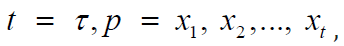the points forming this cycle will obey the following system of equations (11):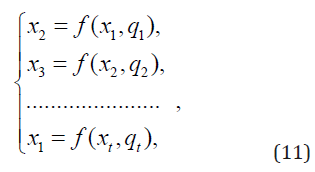To solve the inverse problem, i.e. to find the parameter values at which the map (9) has a given cycle p, it is necessary to express the values from (11) qi. It is clear that not for all possible xi the obtained values of the parameters will satisfy the ratio qi ∈ Q. However, i if this is true for any cycle p = x1, x2,..., xt you can find the values of the parameters q1,q2,..., qt, for which the perturbed map (9) has such a cycle.

If the multiplier of the cycleit’s stable. When among the points forming the cycle, there is a critical xc, the multiplier is always less than one, which ensures stability. In case of unilateral influence of pacemakers on each other, at ε = 0, the display atfor sinusoidal PRC taking into account refractoriness (Figure 8a). The functions f(x), determined from (10), under the mutual influence of oscillators are shown in (Figure 8b) for sinusoidal PRC. Numerical analysis shows that with the growth of ε to a value of ~ 0.5, the area occupied by the resonance zonses becomes larger. This leads to almost complete mixing of languages, so that it is possible to detect zones of different multiplicity in a sufficiently small neighborhood of almost any point (a, γ). However, for these values, self-similar structures are still clearly visible. As the nonlinearity parameter ε increases further, the resonance zones decrease, taking up less and less space. In this case, there is also a very complex picture. Thus, the increase in the force of influence of the oscillators leads to mixing of the initially sufficiently ordered structure in space (a, γ).

Figure 8: Functions f(x) (10) at different h(x) and parameter values. The ratio of periods a is fixed and is 2 (a) h(x) – sinusoidal PRC, ε = 0, γ =0.7, δ =0.5. (b) h(x) is a sinusoidal PRC, ε = 0.5, γ = 0.7, δ =0.5.

Set of values p = x1, x2,..., xt for whom qi ∈ Q and inequalityforms a certain area in the coordinate space R. Each point of this region corresponds to a stable cycle of disturbed mapping. Using the system of equations (11), it is possible to obtain the corresponding region in the parametric space. This enables full control of the system behavior described by the mapping (12). From the point of view of applications to the active environment, this means that a simple parametric impact can control its dynamics. Moreover, if the behavior of the medium is chaotic, with the help of such control it is easy to stabilize it and bring the system to the required dynamic mode of motion . Thus, the main result of this section is quite important for applications: for the systems described by the map (12), there are almost always such external influences, in which such systems will have a prescribed (pre-selected) dynamics.

### The Analogy with Abnormal Heart Rhythms

In conclusion, we draw an analogy between the results and pathological conditions of cardiac tissue. With the help of the constructed models it is possible, for example, to describe the interaction of sinus and ectopic pacemakers, PRC and ABU, and the impact of external disturbances on the sinus rhythm. If, for example, consider the first pulse oscillator PRC, and the second – ABU, it can be found that some stable phase captures correspond to the observed pathologies in clinical practice. In this case, among the various grippers are constructed as normal sinus rhythm (capture ratio 1:1) and classical rhythms Wenkebach (captures multiplicity N:(N-1)) and N:1 AB-blockade. If the first pulse count system of the ABU, and the second ACS, then appear inverted rhythms Wenkebach (similar to direct, but that changes the role of the ventricles and the Atria) observed in some patients. It should be noted that the considered response functions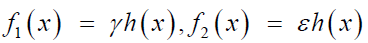for different approximations of the form, the functions h(x) are model. They were taken to analyze the characteristic features of the dynamics of two nonlinear interacting oscillation sources. In practice, these functions should be chosen considering additional physical assumptions about the nature of the interaction and consider the experimental data on the response of a single oscillatory system to single pulses of external perturbation. For example, in  the effect of short pulses on aggregates of spontaneously oscillating cells from the embryo heart was considered. The experimentally obtained phase response curves were approximated by exponential functions, and the “internal” parameters were chosen for the best correspondence of the curve graphs to the experimental points. Their dependence on physical parameters was also chosen in this way. As a result, the phase diagram obtained numerically corresponded well enough to the real dynamics of the system.

Presence of wide areas of phase captures (Figures 4-7), in such systems, various types of synchronization of two oscillators are possible, which qualitatively correspond to some types of cardiac arrhythmias. The phase diagram allows to reveal under what conditions of interaction (i.e. at what values of parameters a, γ, ε and δ) this or that kind of synchronization is possible. Moreover, all the phase patterns presented in this paper indicate that with increasing nonlinearity (i.e., with the growth of the γ parameter), the regions with different captures begin to overlap. Knowledge of such areas and the dynamics of the system in these areas allows by external perturbation (for example, a series of single pulses) to withdraw the system from the unwanted mode of synchronization to a more favorable mode, which is vital. Analysis of phase diagrams makes it possible to find ways to control such systems. We consider the effect of additional periodic pulse action on the behavior of interacting oscillatory subsystems. The study of possible modes of behavior of such a system by varying the frequency and amplitude of the external perturbation will lead its dynamics to a predetermined, for example, to complete suppression of the ectopic sine pacemaker. This problem, considered in the next section, is very relevant for the General theory of control of nonlinear dynamic systems and excitable media, in particular, cardiac tissue, which is satisfactorily described in the models [12,13].

#### Summary

Modern methods of removing the heart from the state of fibrillation are very rigid (supply of a short electrical pulse of a huge voltage and a large current). The development of nonlinear dynamics and Synergetic made it possible to understand that such a force effect is not necessary. Often enough weak electrical effects directly on the heart muscle. Precisely, if there are spiral waves with opposite directions of rotation in the medium, then, choosing the phase and frequency of external action, it is possible to achieve the movement of the centers of the two waves towards each other and their annihilation. Now the word for careful experimental research. The theory of dynamic systems describes many processes inherent in active media, including some types of arrhythmias [1,3]. Since arrhythmias are caused by certain disorders in the heart muscle and, therefore, are pathological conditions, the modeling of such systems is of great practical interest and can bring closer to solving the problem of the possibility of controlling their behavior through external influences. This, in turn, allows us to come close to the problem of soft withdrawal of active systems from the state of developed space–time chaos that characterizes some types of pathologies [14-17]. A noteworthy work is presented in the publication . In this paper, we have implemented a model of the heart, which describes the real behavior of rhythmic and arrhythmic processes in the heart.

Research Article

# Discrete Models of Active Media in Attached to The Activities of Cardiac Arrhythmia

### Sergey Belyakin1* and Sergey Shuteev2

Author Affiliations

1Department of General Physics, Physics Faculty, Russia

2Laboratory of dynamic systems, Physics Faculty, Russia

Received: October 23, 2019 | Published: November 05, 2019

Corresponding author: Sergey Belyakin, Department of General Physics, Physics Faculty, Moscow, Russia

DOI: 10.26717/BJSTR.2019.22.003764

#### Abstract

This publication discusses models that describe the heart tissue as an active conductive system, considering its self-oscillating properties. In this type of models, cardiac rhythms can be described on the basis of the theory of dynamic systems, which justifies the need to build a universal model of oscillating medium. This type of model contributes to the understanding of these pathologies’ cardiac activity as parasecoli and AB-blockade.